We recommend reading the MeanShift vignette Vignette 1 - Clustering via the Mean Shift Algorithm, vignette( "MeanShift-clustering" ), before reading this vignette on clustering for functional data. The previous vignette contains a description of the mean shift algorithm and its use for clustering via the MeanShift package. This vignette assumes some familiarity with the mean shift algorithm and the msClustering and bmsClustering functions of the MeanShift package.

## Functional data

In Functional Data Analysis (FDA) (Ramsay and Silverman 2005; Frédéric Ferraty and Vieu 2006; Frédéric Ferraty and Romain 2011; Horváth and Kokoszka 2012), we think of curves and other functions as the fundamental unit of measurement.

Clustering is an important problem in FDA because it is often of critical interest to identify subpopulations based on the shapes of the measured curves.

## Clustering functional data with the mean shift algorithm

Mode-based clustering, in constrast to many commonly-used clustering methods (e.g. $$k$$-means) has a population formulation (Chacón 2015).

Ciollaro, Genovese, and Wang (2016) show that the population framework of nonparametric mode-based clustering can be extended to the functional setting, despite the fact that function spaces generally lack Lebesgue measure and proper probability densities cannot be easily defined.

From a practical perspective, it is interesting to extend the mean-shift algorithm and use it to cluster functional data. The MeanShift package provides a way to use the mean shift algorithm functions msClustering and bmsClustering with functional data by means of the function projectCurveWavelets.

Given a sample of (possibly noisy) curves $$\{X_1(t_{1,j}),X_2(t_{2,j}),\dots,X_n(t_{n,j})\}$$ observed on a discrete grid $$\{t_{i,j}\}_{j=1}^{m_i}$$, the procedure is as follows:

1. each curve in the sample is projected onto a $$L^2$$ wavelet basis using the DWT (Discrete Wavelet Transform), see Nason (2010)

2. the wavelet coefficients of each curve are thresholded and yield a compressed and sparse representation of each functional datum; the coefficients are arranged by column on a matrix X

3. the mean shift algorithm is applied on X with by means of the function msClustering or bmsClustering; cluster labels and modal coefficients are identified

4. the modal curves/cluster representatives are reconstructed from the modal wavelet coefficients by means of the inverse DWT.

Steps 1, 2, and 4 above are handled with the function projectCurveWavelets.

## Example: clustering signatures data

We illustrate the above procedure by means of an application to a subset of data taken from the Signature Verification Competition (http://www.cse.ust.hk/svc2004/). See also Yeung et al. (2004) and Geenens (2011).

The dataset that we consider contains 5 different types of signatures. For each of these 5 signatures, we observe 20 replications performed by the signature’s owner on a digital tablet1.

For each signature, we observe 7 functional variables:

• x-coordinate: the x coordinate of the pen on the pad
• y-coordinate: the y coordinate of the pen on the pad
• time-stamp: a time stamp
• button status: 0 -> pen is up, 1 -> pen is touching the tablet
• azimuth: clockwise rotation of the cursor about the z-axis
• altitude: angle upward towards the positive z-axis
• pressure: adjusted state of the normal pressure.
## load "MeanShift" package
library( MeanShift )
## load the signatures dataset
ls()
##   "altitude.list"  "azimuth.list"   "bms.clustering" "button.list"
##   "h.cand"         "ms.labels1"     "ms.labels3"     "ms.labels6"
##   "ms.modes1"      "ms.modes3"      "ms.modes6"      "pressure.list"
##  "seeds"          "seeds.data"     "seeds.labels"   "t.list"
##  "tmp.labels3"    "x.list"         "y.list"
## create true signature labels
signatures.labels <- rep( 1:5, rep( 20, 5 ) )

Let’s take a look at some signatures.

## plot some signatures
plot( x.list[], y.list[], type="o", pch=16, main="Type 1 signature", xlab="x", ylab="y" )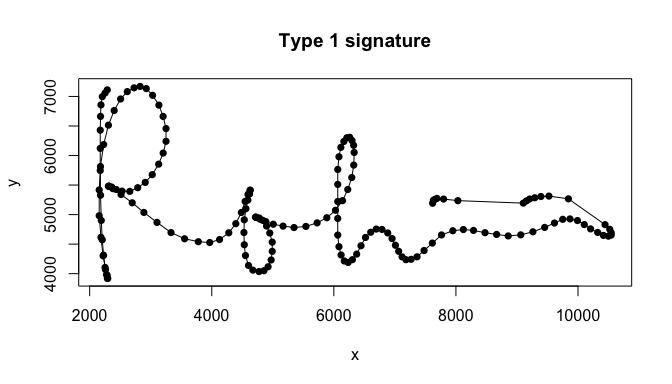plot( x.list[], y.list[], type="o", pch=16, main="Type 2 signature", xlab="x", ylab="y" )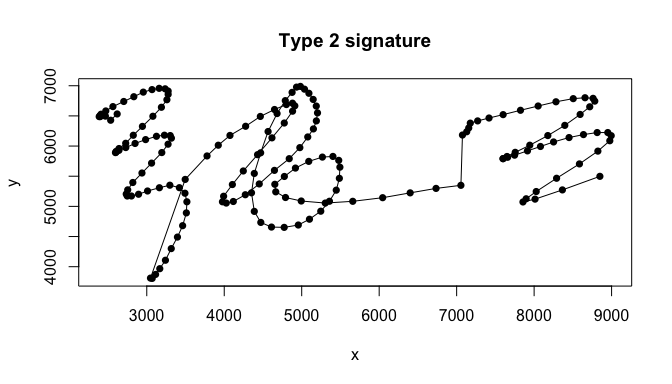plot( x.list[], y.list[], type="o", pch=16, main="Type 3 signature", xlab="x", ylab="y" )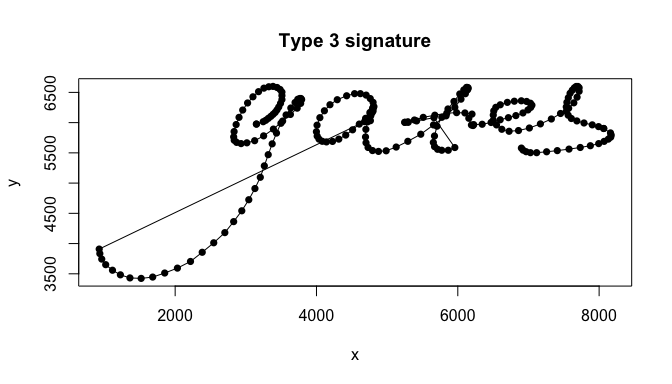plot( x.list[], y.list[], type="o", pch=16, main="Type 4 signature", xlab="x", ylab="y" )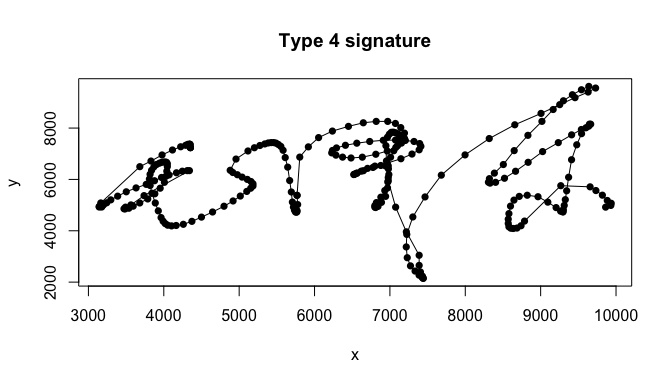plot( x.list[], y.list[], type="o", pch=16, main="Type 5 signature", xlab="x", ylab="y" )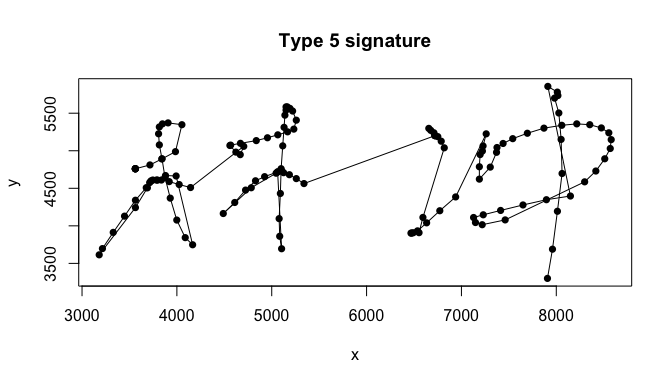Because the number of time-stamps varies across the signatures (though the time-stamps are equispaced for each signature) and the DWT requires that the length of the discretization grid is a positive power of 2, we “jiggle” a little bit the observed curves. In particular, when the discretization grid varies across the curves or its length is not a positive power of 2, projectCurveWavelets “equibalances” all the curves by linearly interpolating them on a common grid whose length is a positive power of 2. For details, see pages 143-150 of Nason (2010), pages 33-34 of Frédéric Ferraty and Vieu (2006), and ?projectCurvesWavelets.

## max grid length across all the signatures
max.grid.length <- max( sapply( t.list, length ) )
print( max.grid.length )
##  410
## we will extend the length of the grid to closest power of 2
grid.length <- 512

The time-stamps in t.list are already standardized between 0 and 1. Before proceding, let us standardize the x coordinates and the y coordinates as well.

## standardize x and y coordinates
standardize <- function( x ){
range <- range( x )
output <- ( x - range ) / diff( range )
return( output )
}
x.list <- lapply( x.list, standardize )
y.list <- lapply( y.list, standardize )

In the next step, we use the function projectCurveWavelets to obtain a wavelet representation of the 7 functional variables for each signature. The functional data in the signatures dataset are very smooth. Furthermore, the process of equibalancing the curves on a grid of 512 points introduces a linear interpolation (which is a form of smoothing) as we explained above. For these reasons, we limit the thresholding of the wavelets to the highest level coefficients only by specifying level=8 in the call to projectCurveWavelets.

## project curves on wavelet basis
wave.x <- mapply( projectCurveWavelets, x=t.list, y=x.list,
MoreArgs=list( grid.length=grid.length, levels=8 ), SIMPLIFY=FALSE )

wave.y <- mapply( projectCurveWavelets, x=t.list, y=y.list,
MoreArgs=list( grid.length=grid.length, levels=8 ), SIMPLIFY=FALSE )

wave.button <- mapply( projectCurveWavelets, x=t.list, y=button.list,
MoreArgs=list( grid.length=grid.length, levels=8 ), SIMPLIFY=FALSE )

wave.azimuth <- mapply( projectCurveWavelets, x=t.list, y=azimuth.list,
MoreArgs=list( grid.length=grid.length, levels=8 ), SIMPLIFY=FALSE )

wave.altitude <- mapply( projectCurveWavelets, x=t.list, y=altitude.list,
MoreArgs=list( grid.length=grid.length, levels=8 ), SIMPLIFY=FALSE )

wave.pressure <- mapply( projectCurveWavelets, x=t.list, y=pressure.list,
MoreArgs=list( grid.length=grid.length, filter.number=4, levels=8 ), SIMPLIFY=FALSE )

We now proceed to write a function to extract the wavelet coefficients from the above objects.

## wavelet coefficients
extractCoefficients <- function( x ){
output <- sapply( x , "[[", "coefficients" )
return( output )
}

Next, we combine all the wavelet coefficients of the 6 features of a signature into a single long vector and we stack these vectors into a matrix.

## combine wavelet objects into a unique list
wave.list <- list( wave.x, wave.y, wave.button, wave.azimuth, wave.altitude,
wave.pressure )

## get coefficients list
wave.coefficients <- lapply( wave.list, extractCoefficients )

## combine coefficients into a unique matrix
wave.coefficients <- do.call( rbind, wave.coefficients )

## 3066 wavelet coefficients: ( 512 - 1 ) * 6 for each one of the 100 signatures
dim( wave.coefficients )
##  3066  100
## note that the matrix is sparse
## print the proportion of non-zero wavelet coefficient for each curve
round( apply( wave.coefficients, 2, function( x ){ mean( x != 0 ) } ), 2 )
## USER1_01 USER1_02 USER1_03 USER1_04 USER1_05 USER1_06 USER1_07 USER1_08
##     0.52     0.52     0.53     0.52     0.51     0.52     0.53     0.52
## USER1_09 USER1_10 USER1_11 USER1_12 USER1_13 USER1_14 USER1_15 USER1_16
##     0.53     0.53     0.53     0.52     0.53     0.53     0.53     0.53
## USER1_17 USER1_18 USER1_19 USER1_20 USER2_01 USER2_02 USER2_03 USER2_04
##     0.53     0.52     0.52     0.52     0.53     0.54     0.54     0.53
## USER2_05 USER2_06 USER2_07 USER2_08 USER2_09 USER2_10 USER2_11 USER2_12
##     0.53     0.53     0.53     0.54     0.54     0.52     0.53     0.54
## USER2_13 USER2_14 USER2_15 USER2_16 USER2_17 USER2_18 USER2_19 USER2_20
##     0.52     0.52     0.52     0.53     0.53     0.54     0.53     0.52
## USER3_01 USER3_02 USER3_03 USER3_04 USER3_05 USER3_06 USER3_07 USER3_08
##     0.54     0.56     0.52     0.54     0.52     0.53     0.52     0.54
## USER3_09 USER3_10 USER3_11 USER3_12 USER3_13 USER3_14 USER3_15 USER3_16
##     0.52     0.53     0.55     0.52     0.53     0.53     0.52     0.52
## USER3_17 USER3_18 USER3_19 USER3_20 USER4_01 USER4_02 USER4_03 USER4_04
##     0.52     0.53     0.52     0.52     0.51     0.51     0.51     0.50
## USER4_05 USER4_06 USER4_07 USER4_08 USER4_09 USER4_10 USER4_11 USER4_12
##     0.50     0.51     0.51     0.51     0.51     0.51     0.50     0.50
## USER4_13 USER4_14 USER4_15 USER4_16 USER4_17 USER4_18 USER4_19 USER4_20
##     0.51     0.50     0.50     0.51     0.51     0.51     0.50     0.50
## USER5_01 USER5_02 USER5_03 USER5_04 USER5_05 USER5_06 USER5_07 USER5_08
##     0.56     0.56     0.56     0.55     0.57     0.55     0.56     0.56
## USER5_09 USER5_10 USER5_11 USER5_12 USER5_13 USER5_14 USER5_15 USER5_16
##     0.55     0.55     0.55     0.55     0.55     0.55     0.55     0.55
## USER5_17 USER5_18 USER5_19 USER5_20
##     0.55     0.55     0.55     0.56
## on average only about 53% of the wavelet coefficients are non-zero

We can now perform clustering using the mean shift algorithm!

## bandwidth candidates
h.cand <- quantile( dist( t( wave.coefficients ) ), seq( 0.03, 0.15, by=0.01 ) )

## clustering using the blurring mean shift algorithm
system.time( clustering <- lapply( h.cand,
function( h ){ bmsClustering( wave.coefficients, h=h ) } ) )
##    user  system elapsed
##  24.571   0.819  31.304

Let’s inspect the clustering of the wavelet coefficients for different bandwidths. The following line of code produces tables with cluster labels and number of signatures associated to each label.

lapply( lapply( lapply( clustering, "[[", "labels" ), table ), sort, decreasing=TRUE )
## $3% ## ## 4 1 8 14 27 29 36 2 3 5 6 7 9 10 11 12 13 15 16 17 18 19 20 21 22 ## 19 18 15 8 3 3 2 1 1 1 1 1 1 1 1 1 1 1 1 1 1 1 1 1 1 ## 23 24 25 26 28 30 31 32 33 34 35 37 38 39 ## 1 1 1 1 1 1 1 1 1 1 1 1 1 1 ## ##$4%
##
##  1  3  7 24 11  2  4  5  6  8  9 10 12 13 14 15 16 17 18 19 20 21 22 23 25
## 19 19 17 10  8  1  1  1  1  1  1  1  1  1  1  1  1  1  1  1  1  1  1  1  1
## 26 27 28 29 30 31 32
##  1  1  1  1  1  1  1
##
## $5% ## ## 1 3 7 21 11 2 4 5 6 8 9 10 12 13 14 15 16 17 18 19 20 22 23 24 25 ## 19 19 17 14 9 1 1 1 1 1 1 1 1 1 1 1 1 1 1 1 1 1 1 1 1 ## 26 27 ## 1 1 ## ##$6%
##
##  3  1  6 18 10 11  2  4  5  7  8  9 12 13 14 15 16 17 19 20 21 22
## 20 19 17 16 10  2  1  1  1  1  1  1  1  1  1  1  1  1  1  1  1  1
##
## $7% ## ## 3 1 6 9 10 2 4 5 7 8 11 12 13 14 15 16 17 18 ## 37 19 17 10 4 1 1 1 1 1 1 1 1 1 1 1 1 1 ## ##$8%
##
##  3  1  6  9 10  2  4  5  7  8 11 12 13 14 15 16
## 39 19 17 10  4  1  1  1  1  1  1  1  1  1  1  1
##
## $9% ## ## 2 1 3 6 7 4 5 8 9 10 11 ## 42 20 17 11 4 1 1 1 1 1 1 ## ##$10%
##
##  2  1  5  3  4  6  7  8  9
## 63 20 11  1  1  1  1  1  1
##
## $11% ## ## 2 1 4 3 5 6 7 8 ## 64 20 11 1 1 1 1 1 ## ##$12%
##
##  1  3  2  4  5  6  7
## 84 11  1  1  1  1  1
##
## $13% ## ## 1 3 4 2 5 6 ## 84 11 2 1 1 1 ## ##$14%
##
##  1  3  4  2  5  6
## 84 11  2  1  1  1
##
## $15% ## ## 1 3 2 4 5 ## 95 2 1 1 1 There appears to be a “persistent” collection of 5-6 clusters across the clustering structures that we obtained along the increasing sequence of bandwidths. Let us examine in more detail the clustering structure associated with the fourth largest bandwidth. index <- 4 ## compare cluster labels and true labels cluster1 <- which( clustering[[index]]$labels == 3 ) # USER2 21:40
print( cluster1 )
##   21 22 23 24 25 26 27 28 29 30 31 32 33 34 35 36 37 38 39 40
print( signatures.labels[cluster1] )
##   2 2 2 2 2 2 2 2 2 2 2 2 2 2 2 2 2 2 2 2
cluster2 <- which( clustering[[index]]$labels == 1 ) # USER1 1:20 print( cluster2 ) ##  1 2 3 4 5 6 7 8 9 10 11 12 13 15 16 17 18 19 20 print( signatures.labels[cluster2] ) ##  1 1 1 1 1 1 1 1 1 1 1 1 1 1 1 1 1 1 1 cluster3 <- which( clustering[[index]]$labels == 6 ) # USER3 40:60
print( cluster3 )
##   43 44 45 46 47 48 49 50 52 53 54 55 56 57 58 59 60
print( signatures.labels[cluster3] )
##   3 3 3 3 3 3 3 3 3 3 3 3 3 3 3 3 3
cluster4 <- which( clustering[[index]]$labels == 18 ) # USER5 80:100 print( cluster4 ) ##  81 82 83 84 85 88 89 90 91 92 94 95 96 97 98 100 print( signatures.labels[cluster4] ) ##  5 5 5 5 5 5 5 5 5 5 5 5 5 5 5 5 cluster5 <- which( clustering[[index]]$labels == 10 ) # USER4 60:80
print( cluster5 )
##   63 64 65 67 71 72 73 74 75 80
print( signatures.labels[cluster5] )
##   4 4 4 4 4 4 4 4 4 4

Finally, we reconstruct the modal signatures from the modal wavelet coefficients.

## modal coefficients for interesting clusters
## (each column corresponds to a feature)
mode1.coeffs <- matrix( clustering[[index]]$components[,3], nrow=511 ) mode2.coeffs <- matrix( clustering[[index]]$components[,1], nrow=511 )
mode3.coeffs <- matrix( clustering[[index]]$components[,6], nrow=511 ) mode4.coeffs <- matrix( clustering[[index]]$components[,18], nrow=511 )
mode5.coeffs <- matrix( clustering[[index]]$components[,10], nrow=511 ) ## put in a list modal.coeffs <- list( mode1.coeffs, mode2.coeffs, mode3.coeffs, mode4.coeffs, mode5.coeffs ) ## we need "wd" objects on which to apply the inverse DWT: ## we can extract the wd object from the wave.xxx lists! wd.x.object <- wave.x[]$y.wdT
wd.y.object <- wave.y[]$y.wdT ## we used all of the 6 features for clustering, but ## for visualization we only care about the projection ## of the functional modes on the x-y plane! ## ## wd.button.object <- wave.button[]$y.wdT
## wd.azimuth.object <- wave.azimuth[]$y.wdT ## wd.altitude.object <- wave.altitude[]$y.wdT
## wd.pressure.object <- wave.pressure[]$y.wdT ## function for inverse DWT invDWT <- function( wd.object, modal.coefficients ){ wd.object$D <- modal.coefficients
output <- wr( wd.object )
return( output )
}

modes.x <- vector( mode="list", length=5 )
modes.y <- vector( mode="list", length=5 )
for( i in 1:5 ){

modes.x[[i]] <- invDWT( wd.x.object, modal.coeffs[[i]][,1] )
modes.y[[i]] <- invDWT( wd.y.object, modal.coeffs[[i]][,2] )

}

## cluster 1
plot( NULL, main="Cluster 1", xlab="x", ylab="y", xlim=c( 0, 1 ),
ylim=c( -0.1, 1.1 ) )
for( i in cluster1 ){

points( x.list[[i]], y.list[[i]], type="o", pch=16, cex=0.5, lwd=0.5 )

}
lines( modes.x[], modes.y[], col=2, lwd=4 )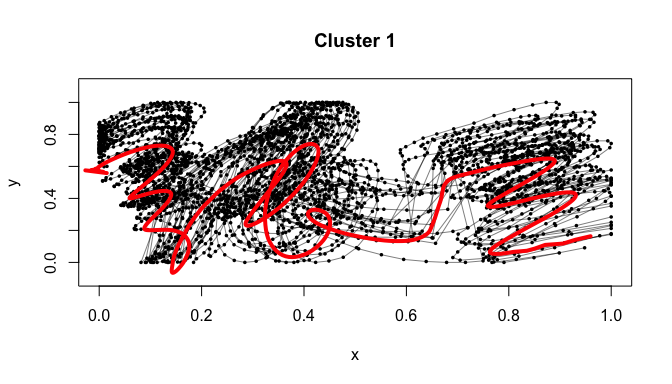## cluster 2
plot( NULL, main="Cluster 2", xlab="x", ylab="y", xlim=c( 0, 1 ),
ylim=c( 0, 1 ) )
for( i in cluster2 ){

points( x.list[[i]], y.list[[i]], type="o", pch=16, cex=0.5, lwd=0.5 )

}
lines( modes.x[], modes.y[], col=2, lwd=4 )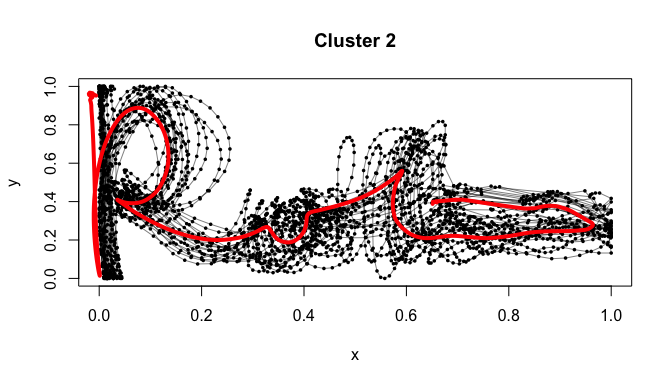## cluster 3
plot( NULL, main="Cluster 3", xlab="x", ylab="y", xlim=c( -0.1, 1 ),
ylim=c( -0.4, 1 ) )
for( i in cluster3 ){

points( x.list[[i]], y.list[[i]], type="o", pch=16, cex=0.5, lwd=0.5 )

}
lines( modes.x[], modes.y[], col=2, lwd=4 )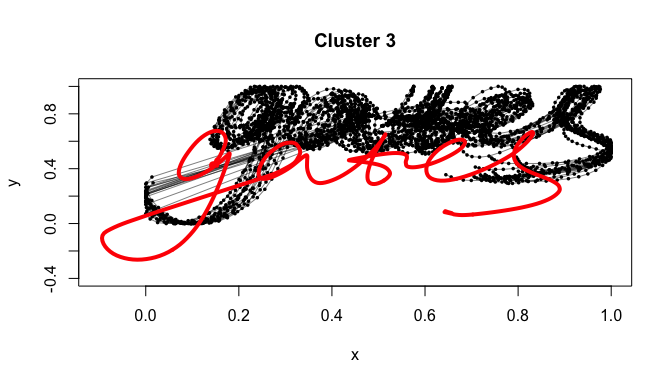## cluster 4
plot( NULL, main="Cluster 4", xlab="x", ylab="y", xlim=c( -0.1, 1 ),
ylim=c( -0.2, 1 ) )
for( i in cluster4 ){

points( x.list[[i]], y.list[[i]], type="o", pch=16, cex=0.5, lwd=0.5 )

}
lines( modes.x[], modes.y[], col=2, lwd=4 )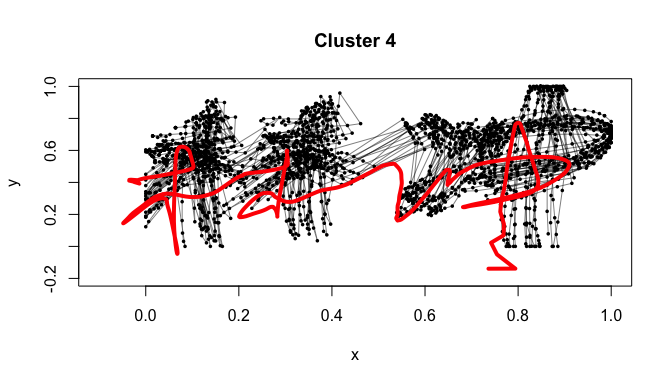## cluster 5
plot( NULL, main="Cluster 5", xlab="x", ylab="y", xlim=c( -0.1, 1.1 ),
ylim=c( -0.1, 1 ) )
for( i in cluster5 ){

points( x.list[[i]], y.list[[i]], type="o", pch=16, cex=0.5, lwd=0.5 )

}
lines( modes.x[], modes.y[], col=2, lwd=4 )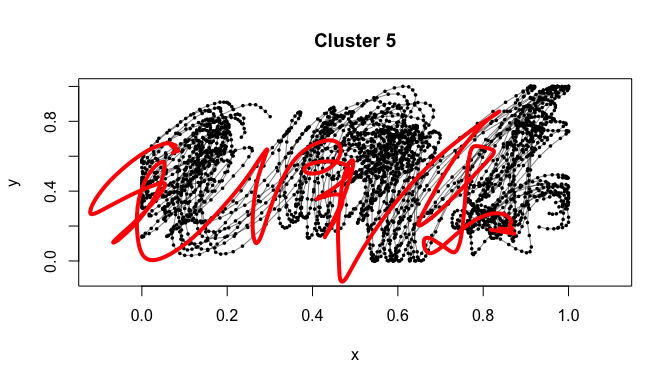Let’s pretend for a moment that we didn’t know that the signatures dataset contains in fact 5 different types of signatures (in a typical clustering problem, the clusters are unknown!). Well, our experiment suggests that by applying the mean shift clustering procedure implemented in the MeanShift package we would have been able to learn the 5 different types of signatures!

By looking at the plots above, it may look surprising at first that the red curves (the modal signatures which are representative of each cluster) are not “centered” in their respective clusters. This shouldn’t be surprising, however. In fact, we performed the mean shift clustering on a 6-dimensional multivariate functional random variable (think a vector whose every entry contains a curve; in our case, the entries were x-coordinate, y-coordinate, button status, azimuth, altitude, and pressure) and then “projected” each 6-dimensional functional mode on a 2-dimensional space to graph the clusters of signatures. While the modes are “centered” in the 6-dimensional space with respect to the 6-dimensional functional representations of the signatures, their projections are not necessarily centered with respect to the projections of the signatures in the 2-dimentional space in which we graphed them!

Chacón, José E. 2015. “A Population Background for Nonparametric Density-Based Clustering.” Statistical Science 30 (4): 518–32.

Ciollaro, Mattia, Christopher R. Genovese, and Daren Wang. 2016. “Nonparametric Clustering of Functional Data Using Pseudo-Densities.” ArXiv Preprint ArXiv:1601.07872.

Ferraty, Frédéric, and Yves Romain. 2011. The Oxford Handbook of Functional Data Analaysis. Oxford University Press.

Ferraty, Frédéric, and Philippe Vieu. 2006. Nonparametric Functional Data Analysis: Theory and Practice. Springer.

Geenens, Gery. 2011. “A Nonparametric Functional Method for Signature Recognition.” In Recent Advances in Functional Data Analysis and Related Topics, edited by Frédéric Ferraty, 141–47. Contributions to Statistics. Physica-Verlag HD.

Horváth, Lajos, and Piotr Kokoszka. 2012. Inference for Functional Data with Applications. Springer.

Nason, Guy. 2010. Wavelet Methods in Statistics with R. Springer Science & Business Media.

Ramsay, James O., and Bernard W. Silverman. 2005. Functional Data Analysis. Springer.

Yeung, Dit-Yan, Hong Chang, Yimin Xiong, Susan George, Ramanujan Kashi, Takashi Matsumoto, and Gerhard Rigoll. 2004. “SVC2004: First International Signature Verification Competition.” In Proceedings of the First International Conference on Biometric Authentication (ICBA), 16–22. Springer.

1. The dataset that we present is obtained by removing the forged signatures from the “Sample Data” dataset available at http://www.cse.ust.hk/svc2004/download.html.